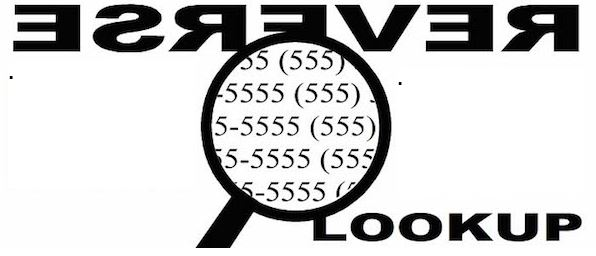# REVERSE LOOKUP IN EXCEL

In my research and tutoring excel, I have found that many people find Reverse lookup concept to be among the top 10 complicated things in excel. This article hopes to shed more light than heat in demystifying the Reverse Lookup in excel.

In the normal lookup, we use the Column and/or Row header to return the value that falls in the intersection. But in the reverse lookup, we shall use the value to return the column and/or row header

For example, say you have below appointments Calendar and you want to lookup &  return the scheduled date & time for customer Joy BellThere are 4 ways to carry out this kind of a Reverse Lookup;

1. ## USING INDEX & SUMPRODUCT

`=INDEX(D3:G3,0,SUMPRODUCT(--(D4:G14=I3)*(COLUMN(D3:G3)-COLUMN(D3)+1)))+INDEX(C4:C14,SUMPRODUCT(--(D4:G14=I3)*(ROW(C4:C14)-ROW(C4)+1)))`

### How it works:

The trick is to break down the formula into small parts;

1. INDEX(D3:G3,0,SUMPRODUCT(–(D4:G14=I3)*(COLUMN(D3:G3)-COLUMN(D3)+1))) this is the part that looks up the Date values in the columns

►SUMPRODUCT(–(D4:G14=I3)*(COLUMN(D3:G3)-COLUMN(D3)+1))  returns a column number given a criteria i.e.

• – -(D4:G14=I3) →returns an array of 1 & 0. 1 represents where criterion is met
`{0,0,0,0;0,0,0,0;0,0,0,0;0,0,0,0;0,0,1,0;0,0,0,0;0,0,0,0;0,0,0,0;0,0,0,0;0,0,0,0;0,0,0,0}`
• (COLUMN(D3:G3)-COLUMN(D3)+1)→ returns an array of relative positions of the columns
` {1,2,3,4}`

SUMPRODUCT takes up the 2 arrays and  using the techniques of conjunction truth table returns 3

►INDEX(D3:E3,0,3) returns the value in column 3 given the range D3:E3= 13-05-2016

2. INDEX(C4:C14,SUMPRODUCT(–(D4:G14=I3)*(ROW(C4:C14)-ROW(C4)+1)) this is the part that looks up the Time values in the rows.

The breakdown of the formula is the same as explained above other than INDEX looks up the rows instead of columns.

When you combine the two parts you form a DateTime Value which you can custom format to fit.

`=INDEX(D3:E3,0,3)+INDEX(C4:C14,5) = 13-05-2016  12:00:00 PM`

## 2.Using Array Formula (INDEX & MAX)

`{=INDEX(D3:G3,MAX((D4:G14=I7)*(COLUMN(D3:G3)-COLUMN(D3)+1)))+INDEX(C4:C14,MAX((D4:G14=I7)*(ROW(C4:C14)-ROW(C4)+1)))}`

### How it works:

1. =INDEX(D3:G3,MAX((D4:G14=I7)*(COLUMN(D3:G3)-COLUMN(D3)+1))) like in the SUMPRODUCT formula, this is the part that looks up the Date Value

(D4:G14=I7)*(COLUMN(D3:G3)-COLUMN(D3)+1) this part generates an array of Column number where the criteria is met and zero for the rest.

`{0,0,0,0;0,0,0,0;0,0,0,0;0,0,0,0;0,0,3,0;0,0,0,0;0,0,0,0;0,0,0,0;0,0,0,0;0,0,0,0;0,0,0,0}`

MAX function returns the  maximum number in the array

`MAX({0,0,0,0;0,0,0,0;0,0,0,0;0,0,0,0;0,0,3,0;0,0,0,0;0,0,0,0;0,0,0,0;0,0,0,0;0,0,0,0;0,0,0,0})=3`

INDEX(D3:E3,0,3) returns the Date Value.

1. INDEX(C4:C14,MAX((D4:G14=I7)*(ROW(C4:C14)-ROW(C4)+1))) this generates the Time Value

The same breakdown works with the Time Value.

NB: This is an Array formula so remember to Ctrl + Shift +Enter

## 3. Using Array Formula (SMALL & IF)

`{=SMALL(IF(D4:G14=I10,(D3:G3+C4:C14)),1)}`

This is the shortest and easiest to understand.

First, you need to understand how Excel stores date and time  i.e. dates as serial numbers and time as a fractional portion of a 24 hour day.

Secondly, you need to understand that a  DateTime Value is just a sum of this Date serial Number and fractional Time Value.

►(D3:G3+C4:C14) returns a two-dimensional array of DateTime Value

`{42501.3333333333,42502.3333333333,42503.3333333333,42504.3333333333;42501.375,42502.375,42503.375,42504.375;42501.4166666667,42502.4166666667,42503.4166666667,42504.4166666667;42501.4583333333,42502.4583333333,42503.4583333333,42504.4583333333;42501.5,42502.5,42503.5,42504.5;42501.5416666667,42502.5416666667,42503.5416666667,42504.5416666667;42501.5833333333,42502.5833333333,42503.5833333333,42504.5833333333;42501.625,42502.625,42503.625,42504.625;42501.6666666667,42502.6666666667,42503.6666666667,42504.6666666667;42501.7083333333,42502.7083333333,42503.7083333333,42504.7083333333;42501.75,42502.75,42503.75,42504.75}`

►IF(D4:G14=I10,(D3:G3+C4:C14)) returns an array of the DateTime value that meets the criteria

`{FALSE,FALSE,FALSE,FALSE;FALSE,FALSE,FALSE,FALSE;FALSE,FALSE,FALSE,FALSE;FALSE,FALSE,FALSE,FALSE;FALSE,FALSE,42503.5,FALSE;FALSE,FALSE,FALSE,FALSE;FALSE,FALSE,FALSE,FALSE;FALSE,FALSE,FALSE,FALSE;FALSE,FALSE,FALSE,FALSE;FALSE,FALSE,FALSE,FALSE;FALSE,FALSE,FALSE,FALSE}`

►SMALL returns the first smallest number in the array =42503.5

►Format the number “dd-mm-yyyy h:mm AM/PM” = 13-05-2016 12:00PM

## 4. Using  AGGREGATE

Other than INDEX & SUMPRODUCT combination this is the other NON-Array formula you can use to do this Reverse lookup

`=AGGREGATE(15,6,((D3:G3+C4:C14)/(D4:G14=I14)),1)`

### How it works;

Watch these Videos on AGGREGATE Function.

## 6 thoughts on “REVERSE LOOKUP IN EXCEL”

1.Deb says:

Hi, would you mind writing out the equation in layman terms please so I can understand the formulae.

1.Crispo Mwangi says:

Hi Deb.
The trick into understanding the formula is knowing how to create an arrary using criteria.

Please watch below videos to understand the AGGREGATE Function first. It will make the the reverse lookup easier to understand.

2.Benson says:

Just came by your blog. It’s amazing and I have it bookmarked for future reference as I like your style of writing. This was a very informative post and really appreciated!

I noticed an error in the post (or maybe I’ve got my eyes crossed while looking through the formulas). In the blog text in the section: “1. USING INDEX & SUMPRODUCT”, you use E[insert row number] as the ending range of your columns whereas the formulas you actually use in the worksheet as well as code snippets is G[insert row number] .

For example:
“►SUMPRODUCT(–(D4:E14=I3)*(COLUMN(D3:E3)-COLUMN(D3)+1)) ”

Mostly cosmetic as most readers will get the crux of the lesson.

I’ll be lurking through your blog as I find this site so resourceful!

Asante bwana. Kazi nzuri kweli!

1.Crispo Mwangi says:

Asante sana Benson!

Thanks for the correction.

3.Peter says:

I do hate direct cell referencing. I wish Dan Bricklin had never invented it!
I think my solution is your solution 3.
I define a named formula ‘appointment’ to refer to the formula
= IF( customer= thisCustomer, date + time )
As a named formula this will always be evaluated as an array and will give all of ‘thisCustomer’s appointment times. The formula on the worksheet
= MIN( appointment )
doesn’t even need to be array entered.

I haven’t checked your formulas but some reverse lookups fail for multiple matches by returning the row index for one and the column index for the other – giving an utter mess.

1.Crispo Mwangi says: